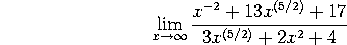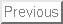This is an actual M403K midterm given on Thursday, September 26, 1991. On that test I allowed a ``crib sheet'' and a non-graphing calculator.

The syllabus has changed some in 9 years, so this exam doesn't cover exactly the same material as our first midterm will. In particular, problem 3 is Chapter 2 material, which will be tested in the second midterm.

Problem 1. Tangent lines

Consider the curve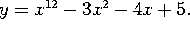Find the equation of the line that is tangent to this curve at the point (1,-1).

Problem 2. Limits and continuity

Consider the function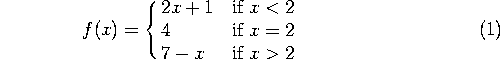a) Find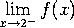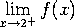, and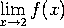, if they exist.

b) Is f(x) continuous at x=2? Why or why not?

Problem 3. Graphs and optimization

Consider the function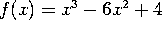.

a) For what values of x is this function increasing? For what values of x is it decreasing?

b) On the interval [-1,3], find the maximum and minimum values of f(x) (and where these values occur).

Problem 4. Taking derivatives

Take the derivatives, with respect to x, of the following functions:

a)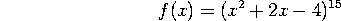b)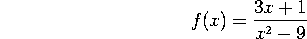c)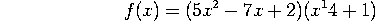d)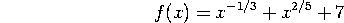Problem 5. Taking limits

Evaluate the following limits:

a)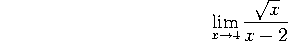b)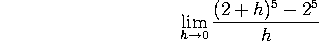c)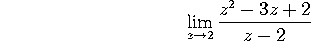d)